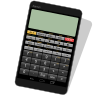# Appsys AMP

Panecal Scientific CalculatorPanecal scientific calculator can display and correct mathematical formulas such as editing and recalculating calculation formulas entered in the past, as well as the capability to use variable memory to only change specific values and do calculations.。
| Get it | Detail | Frequently Asked Questions |

Calculator PanecalSTThe design of the PanecalST is very simple and basic calculator, but the PanecalST has some powerful and useful functions such as cursor formulas editing, calculation of value including tax, percent, last answer, answer history, re-play formulas.
| Get it | Detail |

ShapeInfoThe ShapeInfo is useful application for calculating shape lengths, angles and area. You can get some calculated lengths and angles of the shape from choosing and entering values in the boxes upon the orange points.
| Get it | Detail |